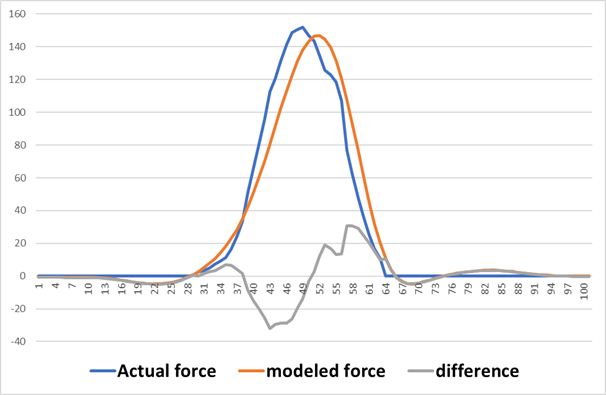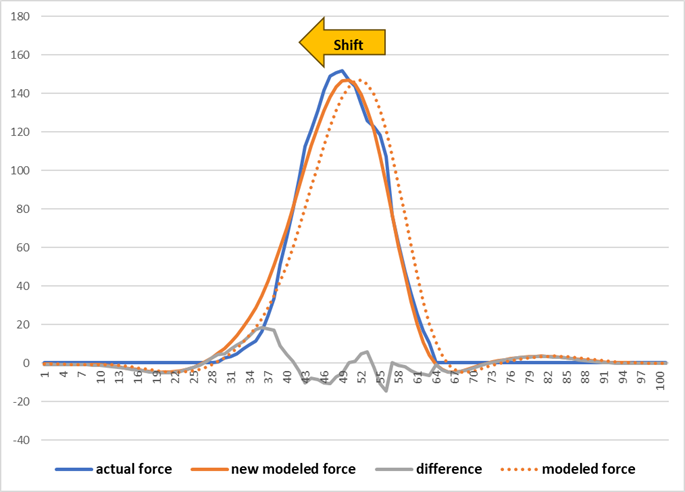# Temporal aspect of AnyForce

Hi Anybody,

I am trying to model an Exosuit that actuates the hip joint. The exosuit has a wire that works as an external muscle, which means shortening this wire pulls the attachment points towards each other. To model this wire, I calculated the wire stiffness using the wire length (the distance between markers at the attachment points) and force in the wire and fitted a 2nd-degree trendline.

I used AnyForce to model the actuation wire. FR1 and FR4 are the markers on the origin and insertion of the wire.

``````AnyForce LateralBand_R = {

AnyKinPLine ExoBand ={
AnyRefNode &Ori = Main.HumanModel.BodyModel.Trunk.SegmentsLumbar.PelvisSeg.ExotendonOrigin_R;
AnyRefNode &Ins = Main.HumanModel.BodyModel.Right.Leg.Seg.Thigh.ExotendonInsertion_R;

AnyDrawPLine Draw = {
Opacity = 1;
Thickness = 0.02;
RGB = {0, 0.9, 1};
};
};

// A + B.x + C.x^2 ...
AnyFloat A = 5824.8;
AnyFloat B = -27745;
AnyFloat C = 33012;

//  // Estimate the length of the band //
AnyVar R14 = vnorm (Main.ModelSetup.C3DFileData.Points.Markers.FR1.PosInterpol(t) - Main.ModelSetup.C3DFileData.Points.Markers.FR4.PosInterpol(t));

AnyFunPolynomial ForceR = {
PolyCoef = {.A, .B, .C};
};

F = -{ForceR(R14)};
};
``````

This is the model result for the wire force compared to the actual force:You can see the difference is considerable. I want to understand if this is the most optimal approach for modeling the wire's force. Also, how can I do this if I want to slightly alter the temporal aspect while modeling the wire's force? What I mean is that the model acts like the newly modeled force, as shown here. In this way, the difference between the modeled and actual forces is lower.Best regards,
Ali

Hi Ali,

I think the problem in the model comes with the way you are modelling the force in the wire. In this way, you are not accounting for the hysteresis in the wire. Shifting the temporal aspect might not be a good idea since it will change for different trials based on the speed.

Instead, I would suggest that you make two interpolation functions, one for the shortening of the wire and the other for the lengthening of the wire. You can also do it, like you have done, by making two polynomial functions. However, an interpolation function would approximate the curve better than a polynomial function. Please read about AnyFunInterpol in the reference manual. AnyFunInterpol needs a parameter value that is monotonically increasing and a unique dependent variable for each parameter value. So, you would need to make two curves. One limitation with interpolation is that you would need some extra data points when you reach the limiting length. You want to be sure that the length of the wire during your trial is within the range of the data you have provided for interpolation.

There is also a previous post that is similar to the problem you want to model. The idea is to use the velocity of AnyKinPLine measure to understand if the wire is shortening or lengthening, and then use the appropriate interpolation function through iffun.

Hopefully, this helps.

Best regards,
Dave